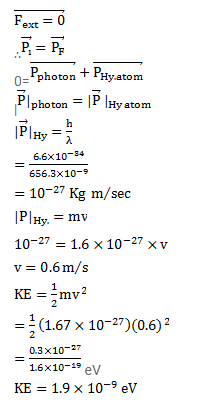# When a photon is emitted by a hydrogen atom,

Question:

When a photon is emitted by a hydrogen atom, the photon carries a momentum with it. Calculate the momentum carried by the photon when a hydrogen atom emits light of wavelength $656.3 \mathrm{~nm}$. With what speed does the atom recoil during this transition? Take the mass of the hydrogen atom $=1.67 \times 10^{-27} \mathrm{~kg}$. Find the kinetic energy of recoil of the atom.

Solution: